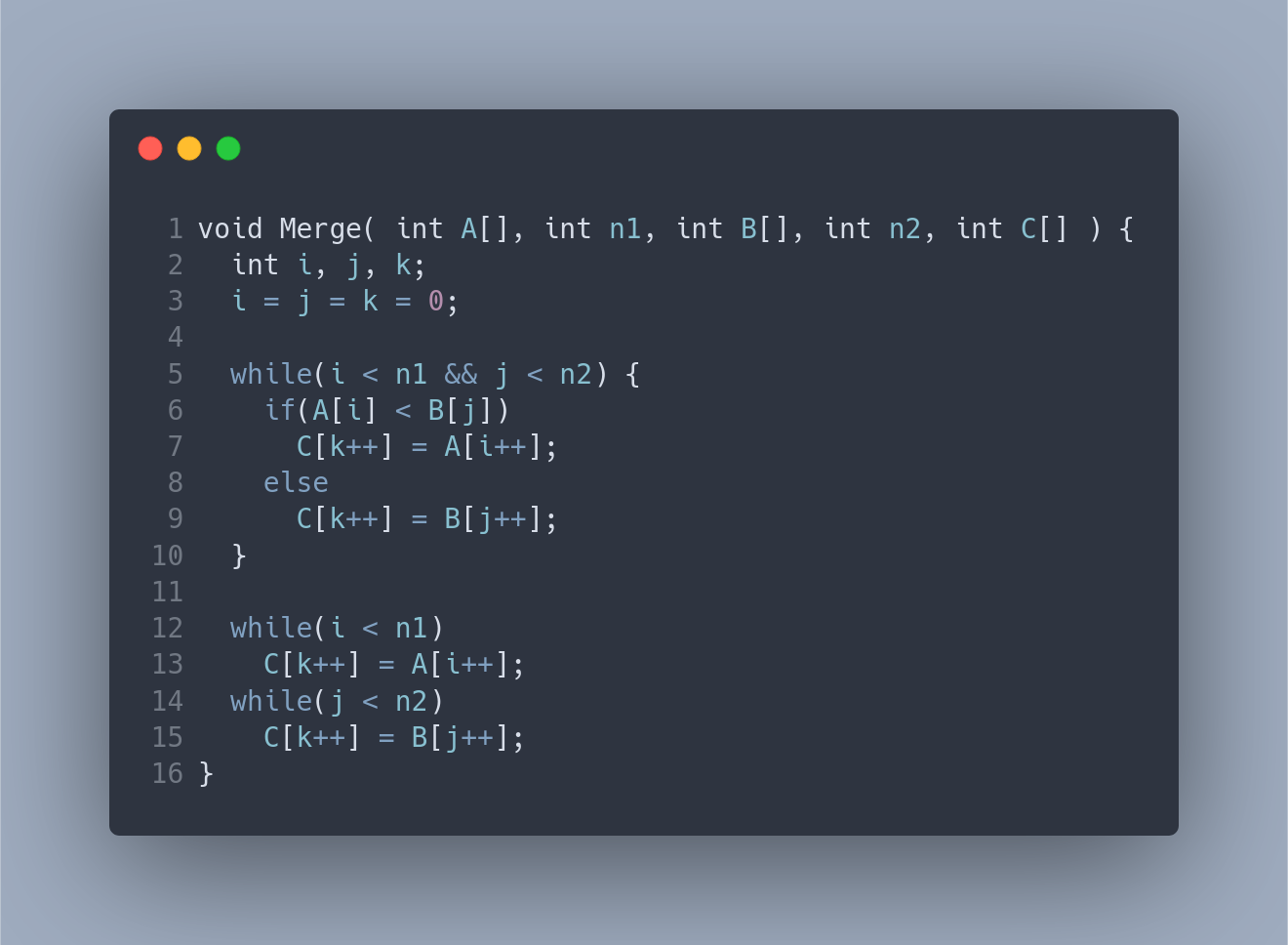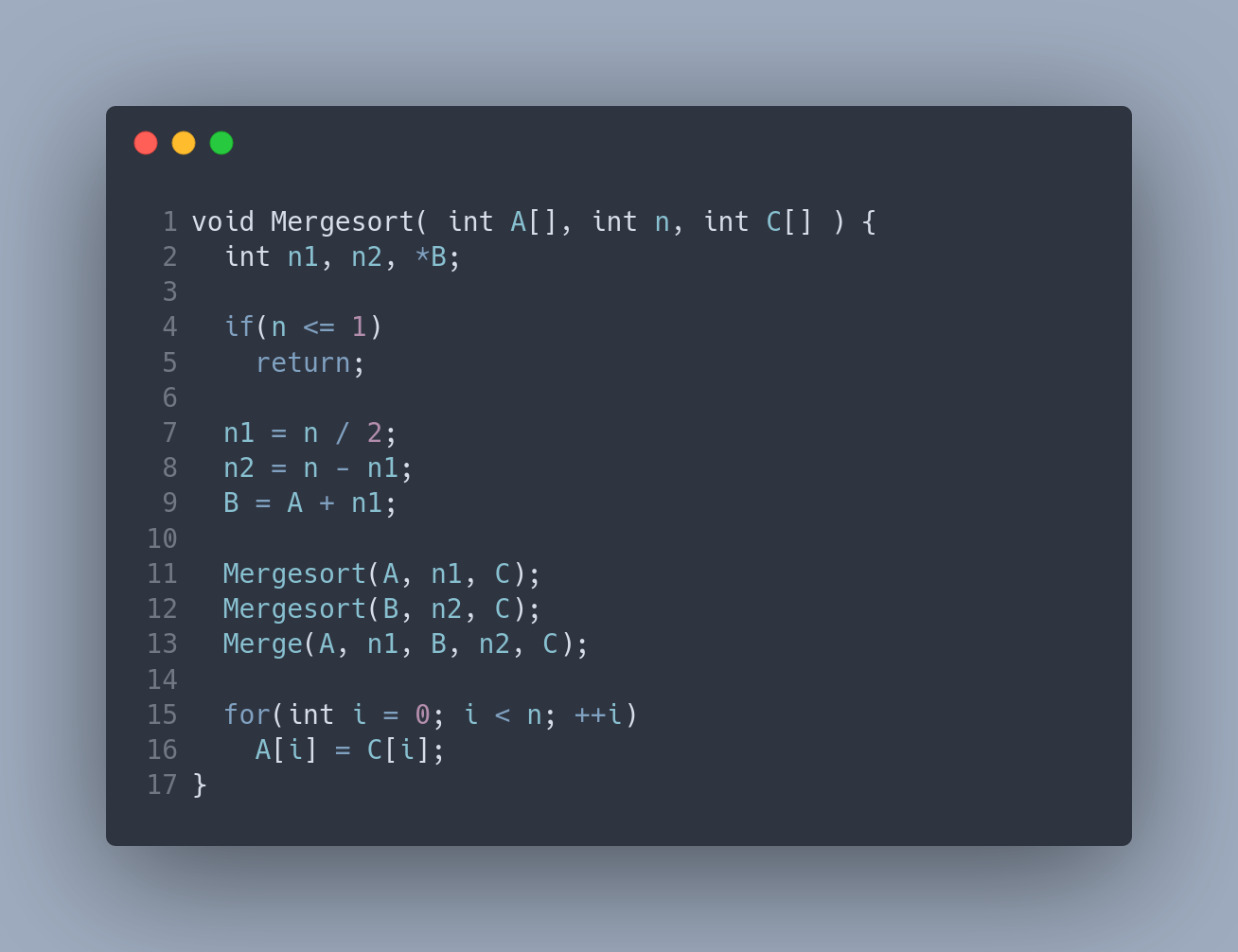# Merge Sort

## Merge Algorithm

This code takes two sorted arrays $$A$$ and $$C$$ and their lengths and creates a sorted array $$C$$. Because the two arrays $$A$$ and $$B$$ are sorted, a sorted array $$C$$ can be created in linear time. The algorithm considers each element from both arrays from the leftmost position and compares these elements to determine which element is to be placed next in the output array. Because both arrays are sorted, the creation of the output array can be done in linear time $$O(n)$$.Let $$M(n_1, n_2)$$ be the number of comparisons that algorithm Merge does on line 6.
$$M(n_1,n_2) \leq n_1 + n_2 -1$$

## MergeSort Algorithm

This code takes a unsorted array $$A$$ and sorts it in ascending order into the array $$C$$. This is done by recursively sorting the left half of the array $$A$$ using $$Mergesort$$, and recursively sorting the right half of the array using $$Mergesort$$. With two sorted subarrays $$A$$ and $$B$$, the $$Merge$$ code can be utilized to create sorted array $$C$$. Because $$Mergesort$$ is an effective use of a divide and conquer algorithm where each subarray is roughly half the size of its parent, the recursive calls to $$Mergesort$$ are made in $$O(log(n))$$ time. For each sorted subarray $$A$$ and $$B$$, the $$Merge$$ algorithm is called and from previous analysis, it is known this algorithm takes $$O(n)$$ time. Therefore, the $$Mergesort$$ algorithm takes a worse case time of $$O(n(log(n)))$$. Let's create and solve the recurrence relation to show this!let $$C(n)$$ be the number of comparisons that $$Mergesort$$ does.
There are $$C(n_1)$$ comparisons for the recursive call on line 11.
There are $$C(n_2)$$ comparisons for the recursive call on line 12.
There are $$n_1 + n_2 - 1$$ comparisons from the call to $$Merge$$ on line 13.

Therefore the recurrence relation is as follows:

$$C(n) \leq C(n_1) + C(n_2) + n_1 + n_2 - 1$$
$$C(n) \leq C(\frac{n}{2}) + C(\frac{n}{2}) + \frac{n}{2} + \frac{n}{2} - 1$$
$$C(n) \leq C(\frac{n}{2}) + n - 1$$

Now solve the recurrence relation.
$$C(n) \leq 2C(\frac{n}{2}) + n - 1$$
$$2C(\frac{n}{2}) \leq 2(2C(\frac{n}{4}) + \frac{n}{2} - 1) = 2^2C(\frac{n}{4}) + n-2$$
$$2^2C(\frac{n}{4}) \leq 2^2(2C(\frac{n}{8}) + \frac{n}{4} - 1) = 2^3C(\frac{n}{8}) + n-4$$
. . .
$$\frac{n}{2}C(2) \leq \frac{n}{2}(2C(1)+(2-1)) = nC(1) + \frac{n}{2}$$
$$nC(1) = 0$$

By adding and cancelling these terms, we get

$$C(n) \leq (n-1) + (n-2) + (n-4) + \dots + (n - \frac{n}{2})$$
$$C(n) \leq \displaystyle\sum_{i=0}^{k-1}n - \displaystyle\sum_{i=0}^{k-1} 2^i$$
$$C(n) \leq kn - (n-1)$$

By our assumption that $$n=2^k$$ and $$k = \log_2n$$

$$C(n) \leq n\log_2n-n+1$$

## Merge Sort Visualizer

Below is a representation of the steps the $$Mergesort$$ algorithm takes. This is shown by ordering columns in ascending order. When the randomize button is invoked, a completely random ordering of columns is generated. These random columns can be sorted by pressing the Merge Sort button. This website shows the steps that the merge sort algorithm takes to sort objects. Enjoy viewing the algorithm and thank you for visiting my merge sort website! I have more interesting projects linked from by website.